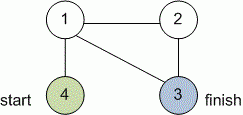Competitions

Given an undirected graph. Find the shortest distance from one given vertex to another.

#### Input

First line contains three positive integers n, s and f (1s, fn100) - the number of vertices in the graph and the number of initial and final vertices. Next n lines describe the adjacency matrix of the graph. If the value of the j-th element of the i-th row is 1, then the graph has a directed edge from vertex i to vertex j.

#### Output

Print the minimum distance from the initial to final vertex. If path does not exist, print 0.Time limit 1 second
Memory limit 128 MiB
Input example #1
4 4 3
0 1 1 1
1 0 1 0
1 1 0 0
1 0 0 0

Output example #1
2

Source SСS - 2011 Sevastopol 08/08/2011 d.2 1st League